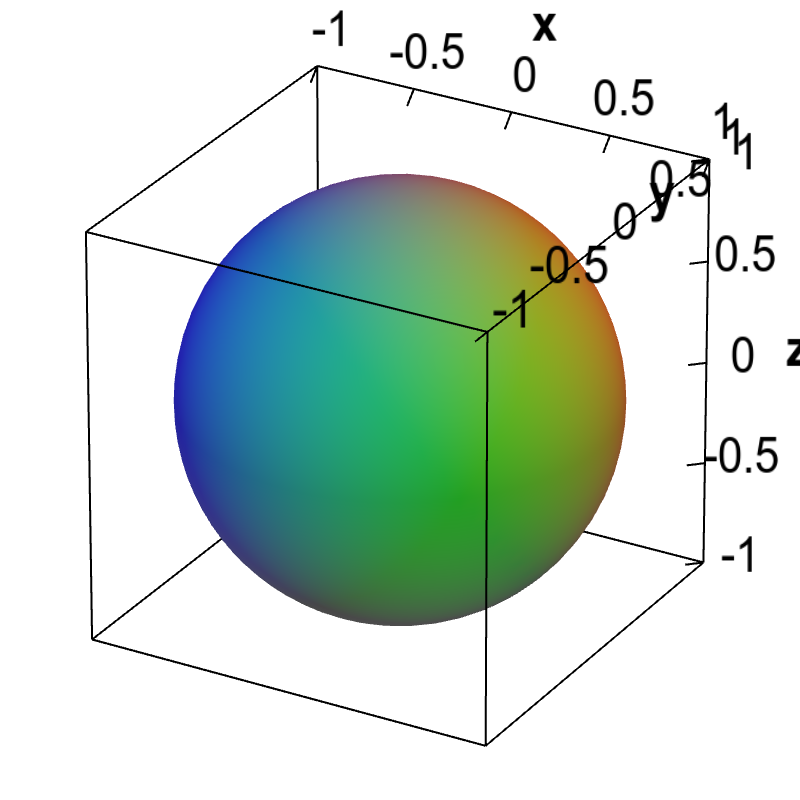# Math Insight

### Applet: A spherical implicit surfaceThe graph of the equation $x^2+y^2+z^2=1$ is the unit sphere centered at the origin.